Courses

RD Sharma Solutions -Ex-21.2 (Part - 2), Surface Area And Volume Of Sphere, Class 9, Maths Notes | Study RD Sharma Solutions for Class 9 Mathematics - Class 9

Class 9: RD Sharma Solutions -Ex-21.2 (Part - 2), Surface Area And Volume Of Sphere, Class 9, Maths Notes | Study RD Sharma Solutions for Class 9 Mathematics - Class 9

The document RD Sharma Solutions -Ex-21.2 (Part - 2), Surface Area And Volume Of Sphere, Class 9, Maths Notes | Study RD Sharma Solutions for Class 9 Mathematics - Class 9 is a part of the Class 9 Course RD Sharma Solutions for Class 9 Mathematics.
All you need of Class 9 at this link: Class 9

Q17. A cylindrical jar of radius 6 cm contains oil. Iron spheres each of radius 1.5 cm are immersed in the oil. How many spheres are necessary to raise the level of the oil by two centimeters?

Sol.

Given that,

Radius of the cylinder jar = 6cm = r1

Level to be rised = 2cm

Radius of each iron sphere = 1.5cm = r2

Numberofsphere =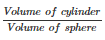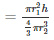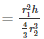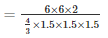Numberofsphere = 16

Q18. A measuring jar of internal diameter 10 cm is partially filled with water. Four equal spherical balls of diameter 2 cm each are dropped in it and they sink down in water completely. What will be the change in the level of water in the jar?

Sol.

Given that,

Diameter of jar = 10cm

Let the level of water be raised by h

Diameter of the spherical bowl = 2cm

Radius of the ball = 1cm

Volume of jar = 4(Volume of spherical ball)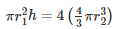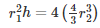5×5×h = 4×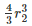5×5×h = 4×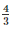×1×1×1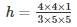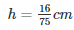Heightofwaterinjar =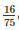cm

Q19. The diameter of a sphere is 6 cm. It is melted and drawn into a wire of diameter 0.2 cm. Find the length of the wire.

Sol.

Given that

Diameter of sphere = 6cm

Radiusofsphere =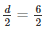= 3cm = r1

Diameterofthewire = 0.2cm

Volume of sphere = Volume of wire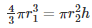=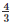×3×3×3 = 0.1×0.1×h

h =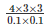h = 3600cmh = 36m

Therefore length of wire = 36m

Q20. The radius of the internal and external surfaces of a hollow spherical shell are 3 cm and 5 cm respectively. If it is melted and recast into a solid cylinder of height 22/3cm. Find the diameter of the cylinder.

Sol.

Given that,

Internal radius of the sphere = 3cm = r1

External radius of the sphere = 5cm = r2

Height of the cylinder =cm = h

Volume of the spherical shell = Volume of cylinder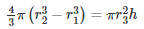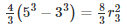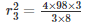r =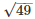r= 7cm

Therefore diameter of the cylinder = 2(radius) = 14cm

Q21. A hemisphere of the lead of radius 7 cm is cast into a right circular cone of height 49 cm. Find the radius of the base.

Sol. Given

Radius of the hemisphere = Volume of cone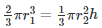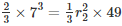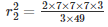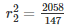r2 = 3.47cm

Therefore radius of the base = 3.74cm

Q22. A hollow sphere of internal and external radii 2cm and 4 cm respectively is melted into a cone of base radius 4cm. Find the height and slant height of the cone.

Sol.

Given that

Hollow sphere external radii = r= 4cm

Internal radii = r1 = 2cm

Height = h

Volume of cone = Volume of sphere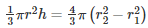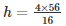h = 14cm

Slantheight(l) =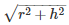Slantheight(l) =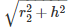l =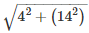l=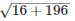l =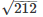l = 14.56cm

Q23. A metallic sphere of radius 10.5 cm is melted and thus recast into small cones, each of radius 3.5 cm and height 3 cm. Find how many cones are obtained.

Sol.

Given that

Metallic sphere of radius = 10.5cm

Let the number of cones obtained be x

vs = x×vcone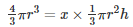x=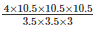x = 126

Therefore number of cones = 126

Q24. A cone and a hemisphere have equal bases and equal volumes. Find the ratio of their heights.

Sol.

Given that

A cone and a hemisphere have equal bases and volumes

vcone = vhemisphere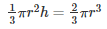r2h = 2r3

h = 2r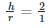h : r = 2 : 1

Therefore the ratio is 2 : 1

Q25. A cone, a hemisphere, and a cylinder stand on equal bases and have the same height. Show that their volumes are in the ratio 1 : 2 : 3.

Sol.

Given that

A cone, a hemisphere and a cylinder stand on one equal bases and have the same weight

We know that

vcone : vhemisphere : vcylinder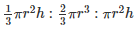multiplying by 3

πr2h : 2πr3 : 3πr2hπr3 : 2πr3 : 3πr(∵ r = h and r2h = r3)

1 : 2 : 3

Thereforetheratiois1 : 2 : 3.

Q26. A cylindrical tub of radius 12 cm contains water to a depth of 20 cm. A spherical form ball is dropped into the tub and thus the level of water is raised by 6.75 cm. What is the radius of the ball?

Sol.

Radius of cylindrical tub = 12cm

Depth = 20cm

Let r be the radius of the ball

Then

Volume of the ball = Volume of water raisedπr3 = πr2h

r3 =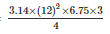r3 = 729

r=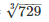r = 9cm

Therefore radius of the ball = 9cm

Q27. The largest sphere is carved out of a cube of side 10.5 cm. Find the volume of the sphere.

Sol.

Side of cube = 10.5cm

Volume of sphere = v

Diameter of the largest sphere = 10.5cm

2r = 10.5

r = 5.25cm

Volumeofsphere =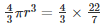×5.25×5.25×5.25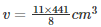v = 606.375cm3

Q28. A sphere, a cylinder, and a cone have the same diameter. The height of the cylinder and also the cone are equal to the diameter of the sphere. Find the ratio of their volumes.

Sol.

Let r be the common radius

Height of the cone = height of the cylinder = 2r

Let

v1 = Volume of sphere =πr3

v= Volume of cylinder = πr2h =  πr2×2r

v= Volume of cone =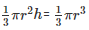Now

v1 : v2 : v3 =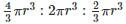= 4 : 6 : 2 = 2 : 3 : 1

Q29. A cube of side 4 cm contains a sphere touching its side. Find the volume of the gap in between.

Sol.

It is given that

Cube side = 4cm

Volume of cube = (4cm)3 = 64cm3

Diameter of the sphere = Length of the side of the cube = 4cm

Therefore radius of the sphere = 2cm

Volume of the sphere =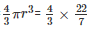×(2)= 33.52cm3

Volume of gap = Volume of cube - Volume of sphere

= 64cm3 - 33.52cm3 = 30.48cm3

Q30. A hemispherical tank is made up of an iron sheet 1 cm thick. If the inner radius is 1 m, then find the volume of the iron used to make the tank.

Sol.

Given that,

Inner radius of the hemispherical tank = 1m = r1

Thickness of the hemispherical tank = 1cm = 0.01m

Outer radius of hemispherical tank = (1+0.01) = 1.01m = = r2

Volume of iron used to make the tank =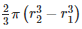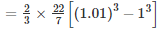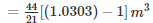= 0.06348m3.

Q31. A capsule of medicine is in the shape of a sphere of diameter 3.5 mm. How much medicine (mm3) is needed to fill this capsule?

Sol.

Given that

Diameter of capsule = 3.5mm

Radius =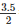= 1.75mm

Volume of spherical sphere =πr3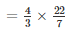×(1.75)3

= 22.458mm3

Therefore 22.46mm3 of medicine is required

Q32. The diameter of the moon is approximately one - fourth of the diameter of the earth. What is the earth the volume of the moon?

Sol.

Diameter of moon =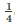thdiameter of earth

Let the diameter of earth be d, so radius =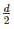Then diameter of moon =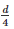Radius =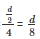Volume of moon =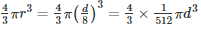Volume of earth =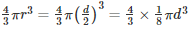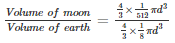=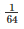Thus the volume of the moon isof volume of earth

The document RD Sharma Solutions -Ex-21.2 (Part - 2), Surface Area And Volume Of Sphere, Class 9, Maths Notes | Study RD Sharma Solutions for Class 9 Mathematics - Class 9 is a part of the Class 9 Course RD Sharma Solutions for Class 9 Mathematics.
All you need of Class 9 at this link: Class 9Use Code STAYHOME200 and get INR 200 additional OFF Use Coupon Code

Top Courses for Class 9RD Sharma Solutions for Class 9 Mathematics

91 docs

Top Courses for Class 9Track your progress, build streaks, highlight & save important lessons and more!

,

,

,

,

,

,

,

,

,

,

,

,

,

,

,

,

,

,

,

,

,

,

,

,

,

,

,

,

,

,

;